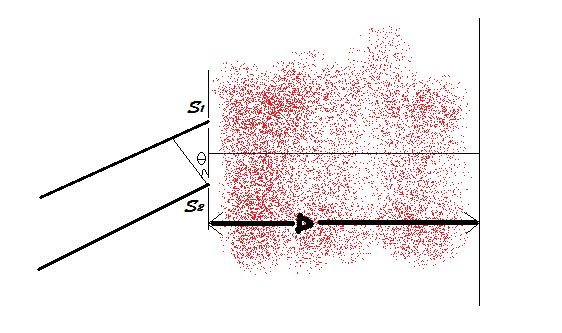# Challenges in optics 4Young's double slit experiment is performed. The distance between two slits is $d$. The distance between slit plane and screen is $D$. Now, there exists a liquid of refractive index $r_{0}$. The refractive index of the liquid is raised with time as per the law: $r=r_{0}+at+bt^{2}$, where $a$ and $b$ are constants. Find the velocity of the central maxima at time $t=5\text{ s}$.

Enter the answer upto 5 decimal places.

Details and Assumptions

• $a=2 \text{ s}^{-1}$, $b=4\text{ s}^{-2}$, $\theta=30^{\circ}$, $D=1\text{ m}$, $r_{0}=6$, $D \gg d$.

• All measurements are in SI units.

×